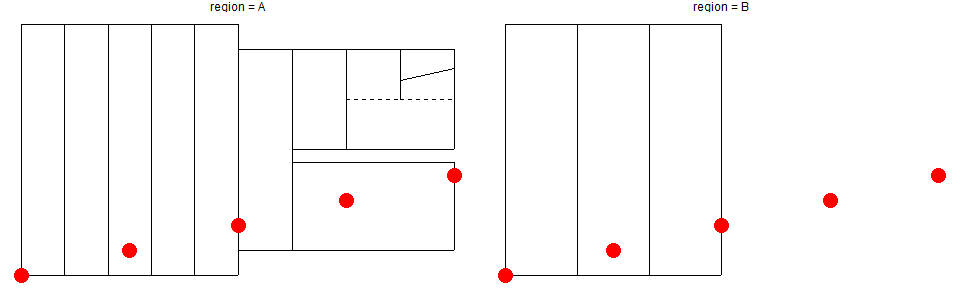# ChronochRt

To execute the examples shown in this vignette, load the package:

library(chronochrt)

## The data set

Chronological data are stored in a table. Each row represents a chron (a chronological unit of any kind). The columns store the information of the chron. The basic information of a chron is:

• Which region is the chron defined for? (This must not necessarily be a geographical region but can also be e.g. a reference or any other kind of overarching category)
• What is its name?
• When does it start?
• When does it end?
• What is its level within the chronological system to be plotted?
• The parameter add.

The parameter add is required for plotting the chart. ChronochRt offers the option to plot an additional chronological column for each region to include e.g. long and short chronologies of regions, or competing chronological systems. Setting the variable add of a chron to TRUE signals ChronochRt to plot this chron in the additional column of the same region.

These six variables are essential for the package and therefore cannot be renamed. When you import or convert a data set, you will have to indicate their corresponding columns in the data set. It is possible to store additional variables in the chrons, such as information to change e.g. the position and angle of the chron’s names or additional information, useful for further work with the data set.

Years BCE are indicated by negative start and end dates, e.g. -100 corresponds to 100 BCE and 100 to 100 A.D., respectively. The package can handle the year 0.

### Important note

In ChronochRt, each chron is independently evaluated. Consequently, all parameters listed above need to be stored for every single chron. It is indispensable that start and end date are identical to the preceding and subsequent chron as well as subchrons starting or ending in the same year. This feature allows the package to plot interruptions and hiatus in the chronological sequence, like the abandonment of settlements or entire regions (see example below). At the same time it simplifies the structure of the chronological data, as each chron stands for itself.

## The chronological chart

This example highlights the structure of a chronological dataset, called chrons, with a x-axis included for educational reasons:

chrons <- add_chron(
region = c("region = A", "region = A", "region = A", "region = A", "region = A", "region = A", "region = A", "region = A", "region = A", "region = A", "region = A", "region = A", "region = B", "region = B", "region = B"),
start = c(-500, -500, -500, -500, -500, -400, -400, 0, 0, "200/200", "200/200", "275_325", -500, -500, -500),
end = c(500, 500, 500, 500, 500, 400, -50, 400, "200/200", 400, "275_325", 400, 500, 500, 500),
level = c(1, 2, 3, 4, 5, 1, 2, 2, 3, 3, 4, 4, 1, 2, 3),
add = c(FALSE, FALSE, FALSE, FALSE, FALSE, TRUE, TRUE, TRUE, TRUE, TRUE, TRUE, TRUE, FALSE, FALSE, FALSE),
new_table = TRUE)

# How does it look like?
print(chrons)
#> # A tibble: 15 x 6
#>    region     name                      start   end     level add
#>    <chr>      <chr>                     <chr>   <chr>   <dbl> <lgl>
#>  1 region = A "level = 1\nadd =\nFALSE" -500    500         1 FALSE
#>  2 region = A "level = 2\nadd =\nFALSE" -500    500         2 FALSE
#>  3 region = A "level = 3\nadd =\nFALSE" -500    500         3 FALSE
#>  4 region = A "level = 4\nadd =\nFALSE" -500    500         4 FALSE
#>  5 region = A "level = 5\nadd =\nFALSE" -500    500         5 FALSE
#>  6 region = A "level = 1\nadd =\nTRUE"  -400    400         1 TRUE
#>  7 region = A "level = 2\nadd =\nTRUE"  -400    -50         2 TRUE
#>  8 region = A "level = 2\nadd =\nTRUE"  0       400         2 TRUE
#>  9 region = A "add =\nTRUE"             0       200/200     3 TRUE
#> 10 region = A "level = 3"               200/200 400         3 TRUE
#> 11 region = A "add = TRUE"              200/200 275_325     4 TRUE
#> 12 region = A "level = 4"               275_325 400         4 TRUE
#> 13 region = B "level = 1\nadd = FALSE"  -500    500         1 FALSE
#> 14 region = B "level = 2\nadd = FALSE"  -500    500         2 FALSE
#> 15 region = B "level = 3\nadd = FALSE"  -500    500         3 FALSE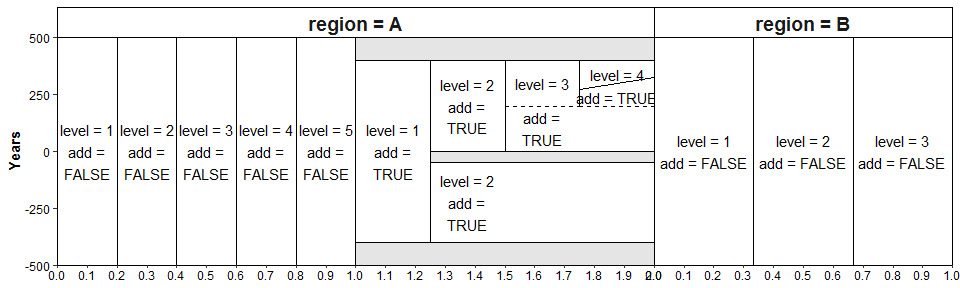Admittedly, the names of the chrons are not pretty but they are intended to show that the information of each chron is directly mirrored in the plot and can be reconstructed from it.

### How does it work?

Each region is plotted independently. Within a region, all chrons with add = FALSE will be evenly scaled according to the maximum level, e.g. in region A in the add = FALSE-column the maximum level is 5, hence, all chrons will be 1/5 wide. Similarly in region B, the maximum levelis 3, consequently all chrons are 1/3 wide. This essentially transform the x-axis into percentages, allowing to easily add additional labels or customise the position and angle of the chrons’ names. Likewise, all chrons in the add = TRUE-column are displayed with their x-value shifted by 1, i.e. they are plotted between 1 and 2.
If not all chrons are further subdivided, empty space is filled by the rightmost chrons. However, by default the names of chrons from the same level will be placed on the same x-value. In complex charts this increases readability and ensures appropriate space for additional labels, even if it can produce odd looking empty space in small plots (see above).

### Unclear start and end dates

Unclear start and end dates can be displayed in two ways: as dashed horizontal lines or as solid diagonal lines. The dashed horizontal lines between two chrons appears when the respective start and/or end date of a chron are coded as "300/300", indicating that they cannot be stated precisely. The diagonal lines are coded with as "300_350" and indicate a transition of a known duration between two periods. The reasons for unclear or vague transitions are manifold. To name a few: the transition between two chronological units or strata are blurred, sites within a region yield different dates for the same transition, transitions are not always clearly expressed in the material record, or it is debated whether this transition exists.
To indicate a transition period, its start and end date are given, e.g. "50/100" or "50_100". For a distinct date, the both years are the same. For example, "100/100" would result in a single horizontal dashed line.
Does the order of the dates matter? Lets play around by using a different region for the different combinations (using arrange_regions() to modify their default alphabetical order in the plot):


name = c("1", "2", "1a", "1b"),
start = c(-100, "50/100", -100, "-25_25"),
end = c("50/100", 200, "-25_25", "50/100"),
level = c(1, 1, 2, 2),
new_table = TRUE) %>%
name = c("1", "2", "1a", "1b"),
start = c(-100, "100/50", -100, "25_-25"),
end = c("100/50", 200, "25_-25", "100/50"),
level = c(1, 1, 2, 2),
new_table = FALSE) %>%
name = c("1", "2", "1a", "1b"),
start = c(-100, "50/100", -100, "-25_25"),
end = c("50/100", 200, "25_-25", "100/50"),
level = c(1, 1, 2, 2),
new_table = FALSE) %>%
name = c("1", "2", "1a", "1b"),
start = c(-100, "100/100", -100, "25_25"),
end = c("100/100", 200, "25_25", "100/100"),
level = c(1, 1, 2, 2),
new_table = FALSE) %>%
arrange_regions(order = c("earlier/later", "later/earlier", "same", "mixed"))

plot_chronochrt(data)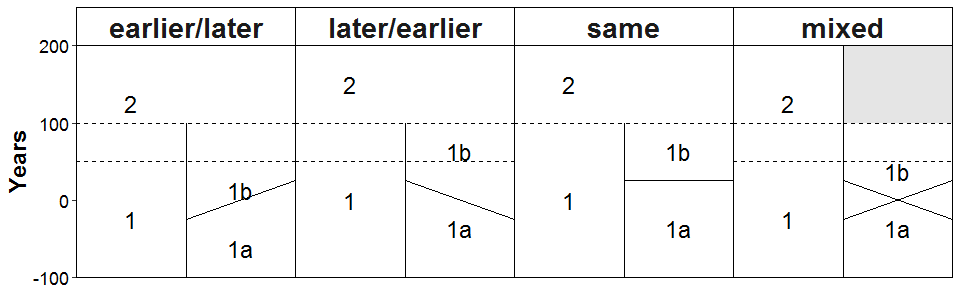Their order matters: Diagonal lines are always drawn from the first to the second value and changing the order will result in a different orientation. Dashed lines do not depend on the order that much. However, consistency matters because mixed orders can result in a failure to recognize the true end of the period. Additionally, the vertical position of the labels are based on the first value.

## Custom labels

Custom labels are optional and hence are stored in an independent data set. Custom text can be placed anywhere on the chronological chart to indicate e.g. special events. As indicated before, it is assumed that they will predominantly appear on the right side of a chronological column, therefore they are right-aligned by default.

Custom labels are recorded with the function add_label_text(). It needs to be specified in which region it should be plotted, the year it should be placed, its position on the x-axis and the label’s text. As the axis scaled from 0 to 1, the value needs to be between 0 and 1 (or 2 if both columns are used):


year = 50,
position = 0.95,
label = "This date in front of the /.",
new = TRUE)

region = "later/earlier",
year = 100,
position = 0.9,
label = "This date in\nfront of the /.",
new = FALSE) %>%
year = 75,
position = 0.75,
label = "Both dates are\nin front of the /.",
new = FALSE)

region = "same",
year = 100,
position = c(0.4, 0.9),
label = "same", new = FALSE)

plot_chronochrt(data, labels_text = text)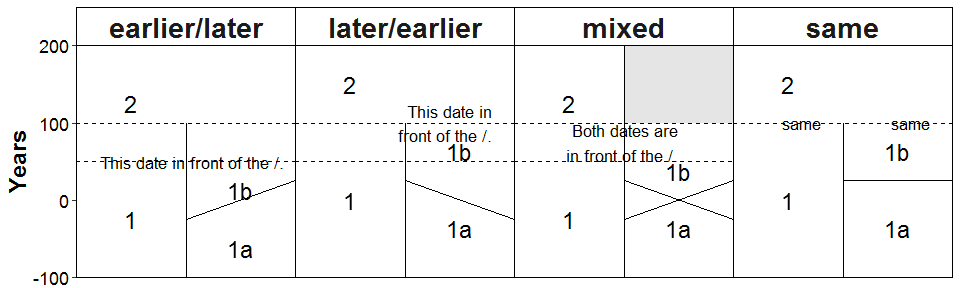Likewise, image labels are added by add_label_images(). This function works exactly the same, with taking the path to the image (web address or path to a file on your computer) instead of the text. It can handle raster and vector files:


year = 50,
position = 0.5,
image_path = "https://www.r-project.org/logo/Rlogo.png",
new = TRUE) %>%
year = 0,
position = 0.5,
image_path = "https://www.r-project.org/logo/Rlogo.svg",
new = FALSE)

plot_chronochrt(data, labels_image = image)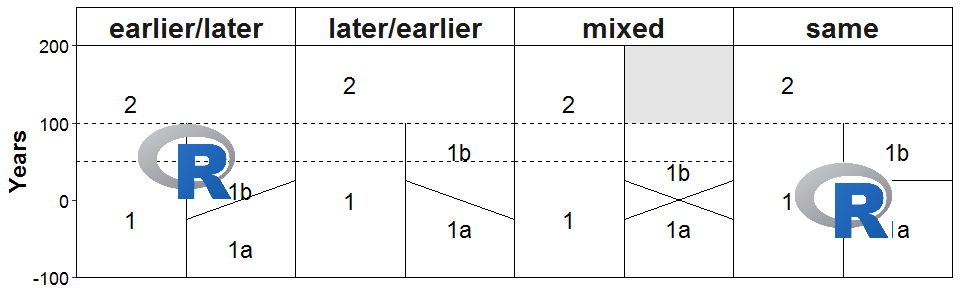## Compatibility with the tidyverse, incl. ggplot2

ChronochRt builds upon the tidyverse environment. Hence its functions can be seamlessly integrated into e.g. pipes (as seen in some examples, look for the pipe operator %>%). Likewise, plot_chronochrt() returns a ggplot2-object, which can be easily enhanced afterwards by e.g. more complex designs using the + operator. As an example, the first plot in this vignette was created with the following code to display the usually omitted x-axis:


plot_chronochrt(chrons, size_chrons = 4, line_break = 20) +
ggplot2::scale_x_continuous(name = NULL, breaks = seq(0, 2, 0.1), minor_breaks = NULL, expand = c(0,0)) +
ggplot2::theme(axis.text.x = ggplot2::element_text(),
axis.ticks.x = ggplot2::element_line())

However, it is strongly recommended to use the geoms provided by ChronochRt for the compilation of more complex design (see chapter “ChronochRt for advanced R users”).

## Some explanation to the example code

The examples shown in this vignette contain some “tricks”, which might facilitate the usage of ChronochRt. They are not features of ChronochRt but of R and the packages ChronochRt builds upon (see above).

• Text wrapping in labels and names of chrons can be easily implemented by typing "\n"instead of a space where the text should be wrapped (spaces at the end of lines will result in unclean text alignment). If the chronological data is imported from an Excel file, text wrapping can be implemented in Excel by inserting line breaks with the combination Alt + Return (Windows) and Control + Command + Enter or Control + Option + Enter (Mac).
• If a value in a column is identical for all rows, it is sufficient to type it in once. It is not necessary to type it for every row (see the code to add the text labels “same”). This facilitates not only coding but also ensures that e.g. the position or text of labels are identical and not erroneous because of typos.

## ChronochRt for advanced R users

Although plot_chronochRt() provides some basic options for the customisation and export of the generated graph, they might not always be sufficient and allows significantly less control how the different elements are placed in the chronological chart and how it is designed. For these reasons, ChronochRt provides two geoms. The geom_chronochRt() will convert the and geom_chronochRtImage(). While geom_chronochRt() transforms a set of chrons into a chronological chart, geom_chronochRtImage() is handling the placement of images. To create a chronological chart as shown in the examples above, they must be combined with a faceting function from and, for the text labels, with the geom_text(), all from the package ggplot2. Consequently, the general code will be similar to:

Putting it into practice to reproduce the last example yields:

plot <- ggplot() +
geom_chronochRt(data = data, mapping = aes(region = region, name = name, start = start, end = end, level = level, add = add)) +
geom_text(data = text, aes(x = position, y = year, label = label)) +
geom_chronochRtImage(data = image, aes(x = position, y = year, image_path = image_path)) +
facet_grid(cols = vars(region))

plot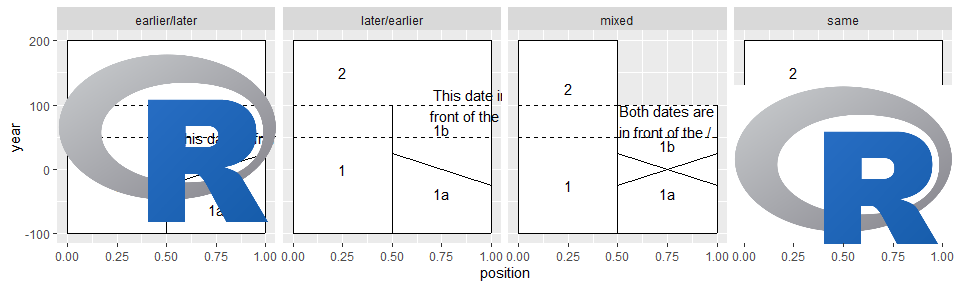Set the general design with the theme_chronochrt():

plot + theme_chronochrt()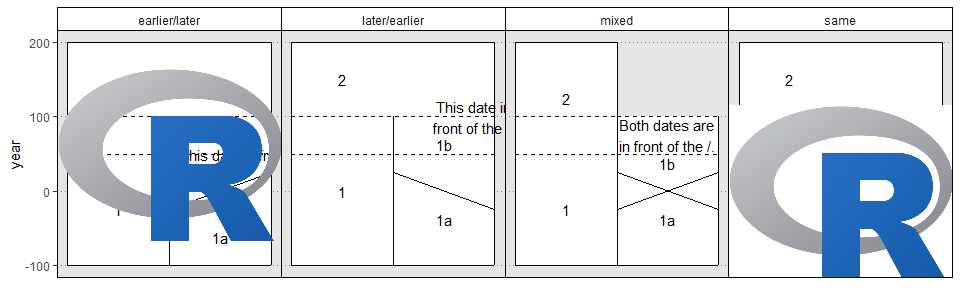However, some fine tuning is still required. By default, the axes are expanded. Additionally, the first example indicates, that the default faceting of ggplot2 is not optimal:

ggplot() +
geom_chronochRt(data = chrons, mapping = aes(region = region, name = name, start = start, end = end, level = level, add = add)) +
facet_grid(cols = vars(region)) +
theme_chronochrt()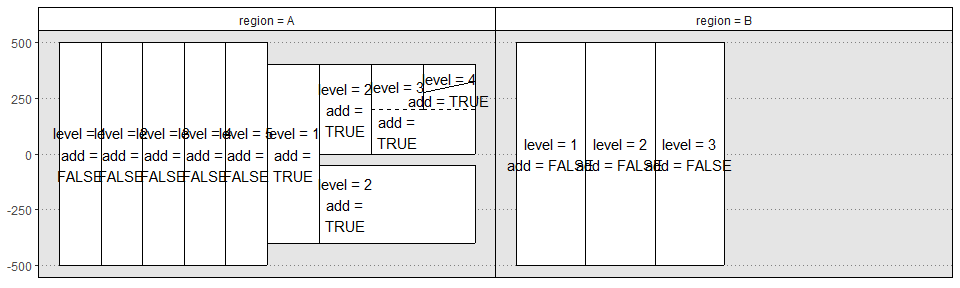Therefore, some additional arguments and functions must be provided to obtain the desired layout:

ggplot() +
geom_chronochRt(data = chrons, mapping = aes(region = region, name = name, start = start, end = end, level = level, add = add)) +
scale_x_continuous(expand = c(0,0)) +
scale_y_continuous(name = "Year", expand = c(0,0)) +
facet_grid(cols = vars(region), scales = "free_x", space = "free_x") +
theme_chronochrt()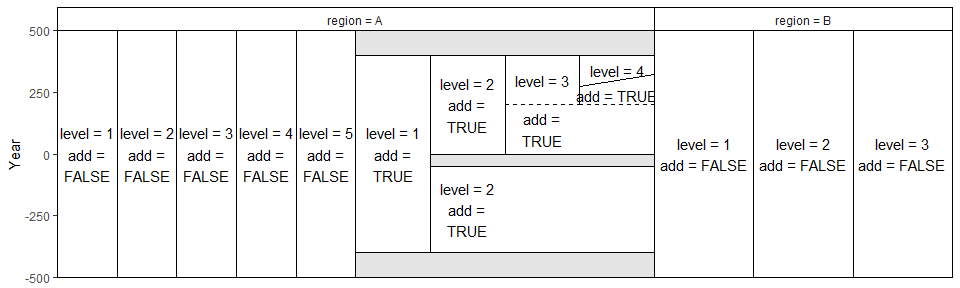And for the example with the image labels, the code now looks like:

ggplot() +
geom_chronochRt(data = data, mapping = aes(region = region, name = name, start = start, end = end, level = level, add = add)) +
geom_text(data = text, aes(x = position, y = year, label = label)) +
geom_chronochRtImage(data = image, aes(x = position, y = year, image_path = image_path)) +
scale_x_continuous(expand = c(0,0)) +
scale_y_continuous(name = "Year", expand = c(0,0)) +
facet_grid(cols = vars(region), scales = "free_x", space = "free_x") +
theme_chronochrt()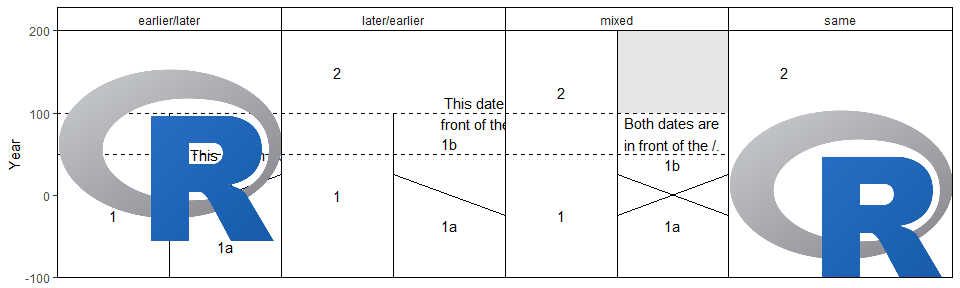Although this approach might seem cumbersome at first, it provides all the freedom for the construction and design of a chronological chart that a regular ggplot2 object offers, like adding other geoms and using other themes:

points <- data.frame(x = seq(0, 2, 0.5),
y = seq(-500,-100, 100))

ggplot() +
geom_chronochRt(data = chrons, aes(region = region, name = NULL, start = start, end = end, level = level, add = add)) +
geom_point(data = points, aes(x = x, y = y), size = 5, colour = "red") +
facet_grid(cols = vars(region), scales = "free_x", space = "free_x") +
theme_void()